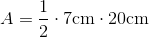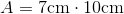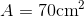# ISEE Upper Level Math : How to find the area of a right triangle

## Example Questions

### Example Question #73 : Geometry

One angle of a right triangle measures 45 degrees, and the hypotenuse measures 8 centimeters. Give the area of the triangle.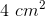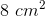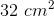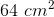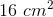Explanation:

This triangle has two angles of 45 and 90 degrees, so the third angle must measure 45 degrees; this is therefore an isosceles right triangle.

By the Pythagorean Theorem, the square of the hypotenuse is equal to the sum of the squares of the other two sides.

Let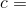hypotenuse and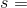side length.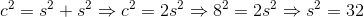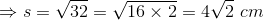We can then plug this side length into the formula for area.### Example Question #74 : Geometry

The legs of a right triangle measure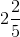and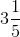. What is its perimeter?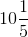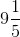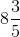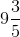Explanation:

The hypotenuse of the triangle can be calculated using the Pythagorean Theorem. Set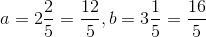: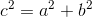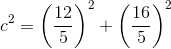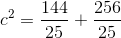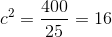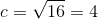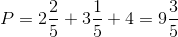### Example Question #75 : Geometry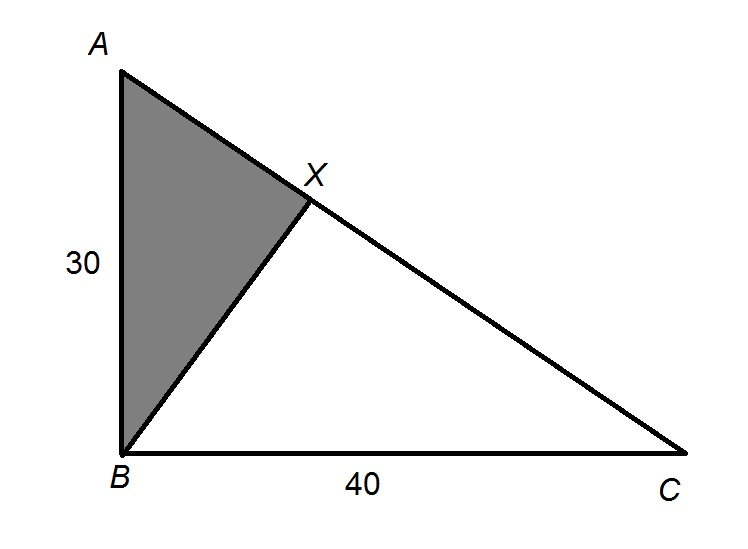Figure NOT drawn to scale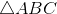is a right triangle with altitude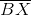. Give the ratio of the area of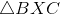to that of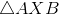.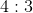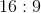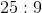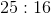Explanation:

The altitude of a right triangle from the vertex of its right angle - which, here, is- divides the triangle into two triangles similar to each other. The ratio of the hypotenuse of the white triangle to that of the gray triangle (which are corresponding sides) is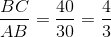,

making this the similarity ratio. The ratio of the areas of two similar triangles is the square of their similarity ratio, which here is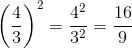, or.

### Example Question #76 : Geometry

Find the area of a right triangle with a base of 7cm and a height of 20cm.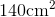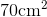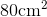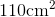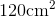Explanation:

To find the area of a right triangle, we will use the following formula: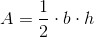where b is the base and is the height of the triangle.

We know the base of the triangle is 7cm.  We know the height of the triangle is 20cm.  Knowing this, we can substitute into the formula.  We get